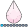## Significance symbol above bars

SAS 9.4

I am attempting to add a second y axis that will enable me to insert an asterisk above significant levels of a variable. I created a data set from proc descript for my estimates and confidence intervals.  I am determining significance by non-overlapping confidence intervals.  Is it possible to create a variable of the levels that are significant from the read in data and use as y= in my second axis to create an asterisk over the significant bar?  Also, is it possible to move the markers up so they are not running into my error bars?

data risk;

set desc_out;

run;

proc template;

define style styles.district;

parent = Styles.htmlblue;

class GraphColors /

'gdata1' = white

'gdata2' = CX4D7EBF

'gdata3' = CX4D7EBF

'gdata4' = CX4D7EBF

'gdata5' = CX4D7EBF

'gdata6' = CX4D7EBF

'gdata7' = CX4D7EBF

'gdata8' = CX4D7EBF;

end;

run;

ods html close;

ods graphics on / width=6.5in height=4in noborder ;

title "Marijuana Use by District, 2015";

footnote "Barchartparm and Scatterplot Overlay for Bar Chart With Error Bars";

options nodate nonumber; /*suppress data stamp and pg #*/

ods html path = "D:\Data" style=styles.district;

ods listing style =styles.district;

proc template;

define statgraph risk ;

begingraph / ;

layout overlay / walldisplay=none /*controls border around graph area */

yaxisopts = (griddisplay = on linearopts = (viewmin = 0 viewmax=20 thresholdmax = 1 )/*grid display*/

offsetmin = 0 offsetmax = 0)/*gets rid of double x axis*/

xaxisopts= (display=( line label tickvalues )/*No ticks*/

label=(" ")/*no bottom legend*/

tickvalueattrs=(size=8pt weight=bold )/*Font size for x axis tick values*/

discreteopts=( TICKVALUEFITPOLICY =split)); /*splits tick values to avoid collision */

barchartparm x=district y=percent / group=district groupdisplay=cluster errorupper=uppct errorlower=lowpct

ERRORBARATTRS= (color=CX4C5D73 thickness=0.5 )

OUTLINEATTRS=(color=gray );

scatterPlot x=district y=percent / dataLabel=percent DATALABELPOSITION=bottomright dataLabelAttrs=(size=9 weight=bold) markerattrs=(size=0);

/* scatterplot x=district y=?var / markerattrs=(size=7 symbol=asterisk color=gray)/*for sig symbol*/;

endlayout;

endgraph;

end;

run;

proc sgrender data=risk template=risk ;

format district district. percent f5.1 ;

run;

ods listing;

ods html close;

4 REPLIES 4

## Re: Significance symbol above bars

Are you using data labels to add the asterisks? That would be my first approach.

Another is the annotate facility but not sure how that interplays with SG Templates.

I'll move this over to the graphing forum where you'll get the SAS graph gurus.

## Re: Significance symbol above bars

You would be better off conducting an actual statistical test. The non-overlapping confidence intervals is an extremely conservative approach to determining statistically significant differences.

## Re: Significance symbol above bars

I understand. But for this particular report, this is the standard procedure for testing for significance.djrisks
Barite | Level 11

## Re: Significance symbol above bars

Hello,

to move the markers up, then you just have to change the value of the y-axis. You can also add just create another yaxis which is y_new = y + 5 for example.

I do not completely understand your other questions. Can you rephrase them please? It will also be useful if you attach your image, and/or the image you would like to produce.

Many thanks,

Kriss

Discussion stats
• 4 replies
• 1665 views
• 0 likes
• 4 in conversation